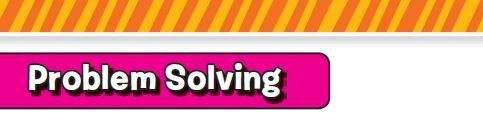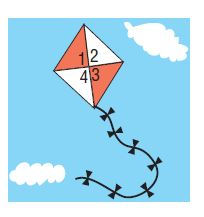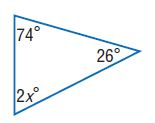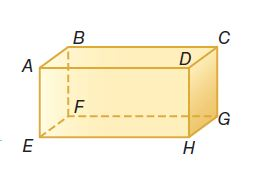Homework Explained - Math Practice 101Dear guest, you are not a registered member. As a guest, you only have read-only access to our books, tests and other practice materials.

As a registered member you can:

Registration is free and doesn't require any type of payment information. Click here to Register.
Go to page:
Chapter 7: Geometric Figures; Chapter Review

###• Question 1

On a trip, Juan bought the kite shown at the right. Identify a pair of vertical angles and adjacent angles.• Type below:
• Question 2

Angle $$\angle Y$$ and $$\angle Z$$ are complementary, and the measure of $$\angle Z$$ is 35°. What is the measure of $$\angle Y$$?

•  $$\angle Y=$$ $$^0$$
• Question 3

Maddie entered her design for an environmental club flag in the school contest. What is the value of x in her flag?• Question 4

The scale on a drawing of a bullfrog is 2 centimeters = 25 millimeters. What is the actual length of the bullfrog if the model length is 11 centimeters?

•  $$\text{mm}$$
• Question 5

Model with Mathematics Draw a top, a side, and a front view of a rectangular table.

• Type below:
• Question 6

Lena is decorating the figure at the right. She is covering the faces with fabric and attaching ribbon to its edges. Identify the figure. Then name the faces and edges.
figure name:
faces:
edges:• Type below:

Yes, email page to my online tutor. (if you didn't add a tutor yet, you can add one here)